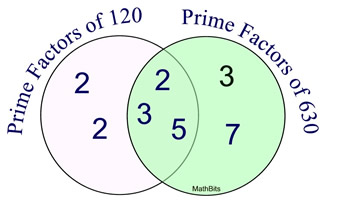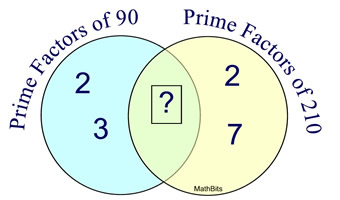Prime Factorization Terms of Use    Contact Person: Donna Roberts1.
Which choice shows the prime factorization of 58?

Choose:
 2 x 2 x 2 x 7 2 x 29 2 x 2 x 14 2 x 5 x 192.
Using the diagram at the right, determine the GCF and LCM for 120 and 630.

Choose:
 GCF = 30     LCM = 2520 GCF = 10     LCM = 24 GCF = 30     LCM = 84 GCF = 30     LCM = 756003.
22 x 3 x 7 x 11 is the prime factorization of what number?

Choose:
 231 462 924 18484.
Which of the following numbers is a composite number?

Choose:
 29 53 91 1215.
Which list shows all the prime numbers greater than 20 and less than 30?

Choose:
 21 and 29 21, 23, and 29 23 and 29 19, 23, and 296.
Hot dogs come in packages of 8. Hot dog buns come in packages of 10. What is the smallest number of packages of each you would need to buy to have exactly one hot dog for every hot dog bun?
Choose:
 4 packages of hot dogs, 5 packages of buns 4 packages of hot dogs, 4 packages of buns 5 packages of hot dogs, 4 packages of buns 5 packages of hot dogs, 5 packages of buns7.
What is wrong with this attempted prime factorization?       36 = 2 x 2 x 9
Choose:
 an exponent must be used 9 is not a prime number 36 is a prime number there is nothing wrong8.
What prime factor(s) belong(s) in the box in the center of this diagram?

Choose:
 5 only 3 only 3 and 5 2 and 39.
Which of the following is a prime factorization?

Choose:
 40 = 5 x 8 21 = 3 x 7 81 = 92 180 = 24 x 1510.
Government intelligence agencies have discovered that encryption techniques based on prime numbers can currently not be cracked. Such techniques can be used to encrypt emails based upon the product of two very large prime numbers. One of the largest primes ever found has over 2 million digits, with new numbers still being found.
Consider a very simple encryption using the product 143. What two prime numbers were multiplied to give this value?

Choose:
 7 and 21 41 and 3 9 and 13 11 and 13